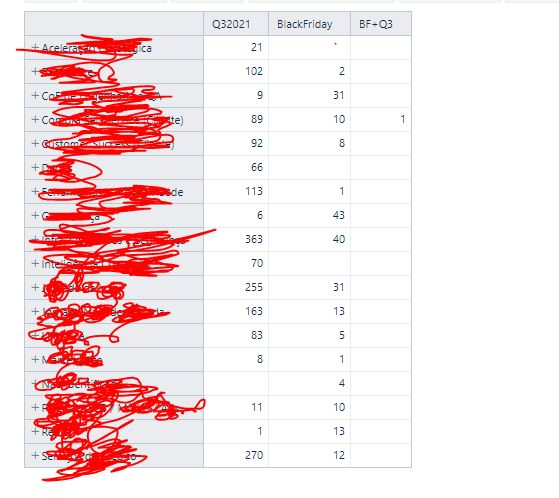# Calculated two kind of labels

I need help with creating a calculated that has the label “Q32021”
AND one or all of the following labels:
LL_BF2021,
BF21TESTE,
BF21_GESTAO,
BF21_POS,
BF20_POS,
LL_BF20,
BF21

I created a calculated member:
[measures].[BlackFriday]

([Label].[Label].[LL_BF2021] +
[Label].[Label].[BF21TESTE] +
[Label].[Label].[BF21_GESTAO] +
[Label].[Label].[BF21_POS] +
[Label].[Label].[BF20_POS] +
[Label].[Label].[LL_BF20] +
[Label].[Label].[BF21])

And another calculated member:
[measures][Q32021]
([Epic Label].[Label].[Q32021])

And finally I created this one thar represents an issue that have one of BlackFriday labels PLUS Q32021 based in this topic:

[measures].[BF+Q3]
NonZero(
Count(
Filter(
Descendants([Issue].CurrentHierarchyMember,[Issue].[Issue]),
(
[Measures].[BlackFriday]
)>0
AND
(
[Measures].[Q32021]
)>0
)
)
)

But when I run my report is getting too slow, with more one minute of execution

Is there a better day to make this calculation?Hi @guinevere,

The measure is the core element of each report to bind data together from different perspectives. When building a calculated measure (in Measures), you might want to refer to some predefined measure to make the calculation work. On the other hand, you might want to group dimension members (labels) by crating calculated members in the dimension (Label dimension) itself.

To make this report work predictably, you might want to update the calculations to match ground rules: Calculated measures and members - eazyBI for Jira.

1. In the "Label" dimension, define a new calculated member “BlackFriday”, to group several labels of interest. Use function Aggregate() to group labels of interest.

``````Aggregate({
[Label].[LL_BF2021],
[Label].[BF21TESTE],
[Label].[BF21_GESTAO],
[Label].[BF21_POS],
[Label].[BF20_POS],
[Label].[LL_BF20],
[Label].[BF21]
})
``````
2. In Measures, update the calculated measure “BlackFriday”. Use a tuple of predefined measure “Issue created count” and calculated member “BlackFriday” to counts how many issues have some label of the “BlackFriday” label list.

``````( [Measures].[Issues created count],
[Label].[BlackFriday] )
``````
3. From the screenshot I see that you have imported the issue link field dimension “Epic Label”.
In Measures, update the calculated measure “Q32021” using a tuple of measure “Issue created count” and specific Epic Label.

``````( [Measures].[Issues created count],
[Epic Label].[Q32021] )
``````
4. In Measures, update the calculated measure “BF+Q3”. Current expressions with functions Count() and Descendants() might be quite slow as it goes through all issues and checks their labels. But you can use tuples again to get the results much faster.

``````( [Measures].[Issues created count],
[Label].[BlackFriday] )
+
( [Measures].[Issues created count],
[Epic Label].[Q32021] )
-
--removes duplicated issues amtchin both BF and Q3
( [Measures].[Issues created count],
[Label].[BlackFriday],
[Epic Label].[Q32021] )
``````

Thre are more details on tuples and calculated measures: Calculated measures - eazyBI for Jira.

Best,
Zane / support@eazyBI.com